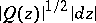# Extremal metric, method of the

(diff) ← Older revision | Latest revision (diff) | Newer revision → (diff)

One of the fundamental methods in geometric function theory, closely connected with differential geometry and topology. The method of the extremal metric is based on the relations between the length of curves belonging to specific homotopy classes and the areas of the domains filled out by them. Here these curves and areas are computed in a special metric corresponding to the peculiarities of the extremal problem under investigation. (About extremal problems in geometric function theory see Univalent function.)

The method of the extremal metric has various forms. The original one is Grötzsch's strip method, which is an essential refinement of the arguments connecting length and area, operating with the characteristic conformal invariants of doubly-connected domains and quadrangles (see Grötzsch principle). Using his strip method, H. Grötzsch obtained a number of classical results in the theory of conformal and quasi-conformal mappings (see, for example, Grötzsch theorems).

The most important stages in the development of the method of the extremal metric were the introduction by L.V. Ahlfors and A. Beurling of the concept of the extremal length of a family of curves, the generalization proposed by J.A. Jenkins of the concept of the modulus of a family of curves to the case of several families of curves, and the proof of the uniqueness of the extremal metric in the moduli problem in this case.

Between 1939 and 1941 O. Teichmüller stated (without proof) a general principle asserting that the solutions of extremal problems in geometric function theory are connected in a definite way with certain quadratic differentials (cf. Quadratic differential). One of the most significant results in the development of the method of the extremal metric was Jenkins' "general coefficient theorem" (see Jenkins theorem and ). This theorem contains as particular applications almost-all known elementary results concerning univalent functions (see, for example, Univalent function). The uniqueness result in Jenkins' theorem was sharpened and an analogue of this theorem was established for quadratic differentials without multiple poles (see ). In this way, certain extremal problems for domains with two and three distinguished boundary components were solved and the set of all extremal mappings was fully analyzed (see  and ). The solution of extremal problems by means of the "general coefficient theorem" is one of the forms of the method of the extremal metric and is much used in research.

Another widely applied form of the method of the extremal metric is the version known as the moduli method. This technique is based on establishing a direct connection between a given extremal problem and a certain moduli problem for one or several families of curves (see Extremal length of a family of curves), and on solving this extremal-metric problem. As a rule, the extremal metric of the relevant moduli problem turns out to be a metric, whereis a quadratic differential the poles of which are determined from the conditions of the given problem. A direct examination of moduli problems for families of curves led to definite results in a number of questions related to the extremal partition of a given domain into a family of simply-connected and doubly-connected domains associated with specific homotopy classes of curves. The formulation of the latter goes back to the studies of M.A. Lavrent'ev and G.M. Goluzin (see ).

The combination of the method of the extremal metric with variational methods and the symmetrization method has also been successful. Thus, the simultaneous use of the moduli method and the method of internal variations (cf. Internal variations, method of) led to the proof of the existence of an extremal metric for the moduli problem for families of curves under very general assumptions. The combination of the method of the extremal metric and the method of symmetrization makes it possible in a number of cases to establish that the distribution of the poles of the associated quadratic differential has certain symmetry, and thus to reduce the given problem to a simpler case.

There are also various other forms of the method of the extremal metric. One of them consists in solving extremal problems both for univalent and for multivalent mappings (in the latter case Teichmüller's principle is not applicable) by making direct use of the expressions for the areas of the images of some subsets of the given domain under the mapping in question, interpreted as the areas of the original sets in some new metric, in terms of the areas of the sets themselves. This form of the method of the extremal metric has turned out to be particularly effective in the solution of problems concerning the span of a multiply-connected domain (see ). A development of this approach is Jenkins' "special coefficient theorem" (see Jenkins theorem).

In a particular case this theorem reduces to the statement of the area principle.

How to Cite This Entry:
Extremal metric, method of the. Encyclopedia of Mathematics. URL: http://encyclopediaofmath.org/index.php?title=Extremal_metric,_method_of_the&oldid=16409
This article was adapted from an original article by G.V. Kuz'mina (originator), which appeared in Encyclopedia of Mathematics - ISBN 1402006098. See original article